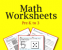Practice makes perfect! Copy the right equation first.

These practice Math Worksheets offer the basic Math facts three times each. We also include our Math flash cards of all four Math operations. Print and reprint as often as your students need practice with our printable Math worksheets.

These practice Math Worksheets offer practicing the basic Math facts three times each. We also include our Math flash cards of all four Math operations. Print and reprint as often as your students need practice! There are 945 pages of printable Math worksheets.

These cover many of the basic math operations like:

• Shapes Worksheets
• Subtraction Worksheets
• Multiplication Worksheets
• Division Worksheets
• And More!

#### Affiliates

Like this product?

Spread the word about it and earn 40.00% of the purchase price on sales you refer.